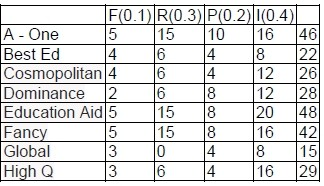### CAT 2018 - Slot 2 - Logical Reasoning and Data Interpretation -An agency entrusted to accredit colleges looks at four parameters

##### An agency entrusted to accredit colleges looks at four parameters: faculty quality (F), reputation (R), placement quality (P), and infrastructure (I). The four parameters are used to arrive at an overall score, which the agency uses to give an accreditation to the colleges. In each parameter, there are five possible letter grades given, each carrying certain points: A (50 points), B (40 points), C (30 points), D (20 points), and F (0 points). The overall score for a college is the weighted sum of the points scored in the four parameters. The weights of the parameters are 0.1, 0.2, 0.3 and 0.4 in some order, but the order is not disclosed. Accreditation is awarded based on the following scheme: Range Accreditation Overall score ≥ 45 AAA 35 ≤ Overall score < 45 BAA 25 ≤ Overall score < 35 BBA 15 ≤ Overall score < 25 BBB Overall score < 15 Junk Eight colleges apply for accreditation, and receive the following grades in the four parameters (F, R, P, and I):   F R P I A-one A A A B Best Ed B C D D Cosmopolitan B D D C Dominance D D B C Education Aid A A B A Fancy A A B B Global C F D D High Q C D D B It is further known that in terms of overall scores: 1. High Q is better than Best Ed; 2. Best Ed is better than Cosmopolitan; and 3. Education Aid is better than A-one.

Q. 1: What is the weight of the faculty quality parameter?
1. 0.3
2. 0.2
3. 0.4
4. 0.1

##### Q. 2: How many colleges receive the accreditation of AAA?

Q. 3: What is the highest overall score among the eight colleges?

Q. 4:How many colleges have overall scores between 31 and 40, both inclusive?
1. 1
2. 3
3. 0
4. 2

Let a, b, c and d be the weights of parameters F, R, P and I respectively. Given,

(i) 30a + 20b + 20c + 40d > 40a + 30b + 20c + 20d (ii) 40a + 30b + 20c + 20d > 40a + 20b + 20c + 30d

(iii) 50a + 50b + 40c + 50d > 50a + 50b + 50c + 40d From (i), 2d > a + b

From (ii), b > d From (iii), d > c

b > d > c

a, b, c and d are 0.1, 0.2, 0.3 and 0.4 in any order.

d cannot be 0.1 or 0.2. (∵ 2d cannot be greater than a + b) d can be 0.3 or 0.4, but given b > d.

b = 0.4, d = 0.3

2(0.3) > 0.4 + a

a < 0.2

a = 0.1, c = 0.2Question 1
Weight of faculty parameter is 0.1. Ans : 0.1

Question 2
Three colleges received AAA rating. Ans : 3

Question 3
Height overall score among the eight colleges is 48. Ans : 48

Question 4
No college has score between 31 and 40 (both inclusive). Ans : 0
##### Checkout Other Questions of CAT 2018 Slot 2 Paper:

Verbal Ability :              |   Q.01- Q.05  |  Q.06- Q.9  |  Q.10- Q.14  |  Q.15- Q.19  |  Q.20- Q.24  |  Q.25- Q.29  |  Q.30 – Q.34  |

Logical Reasoning :    |   Q.29 – Q.32  |

Quantitative Aptitude: |